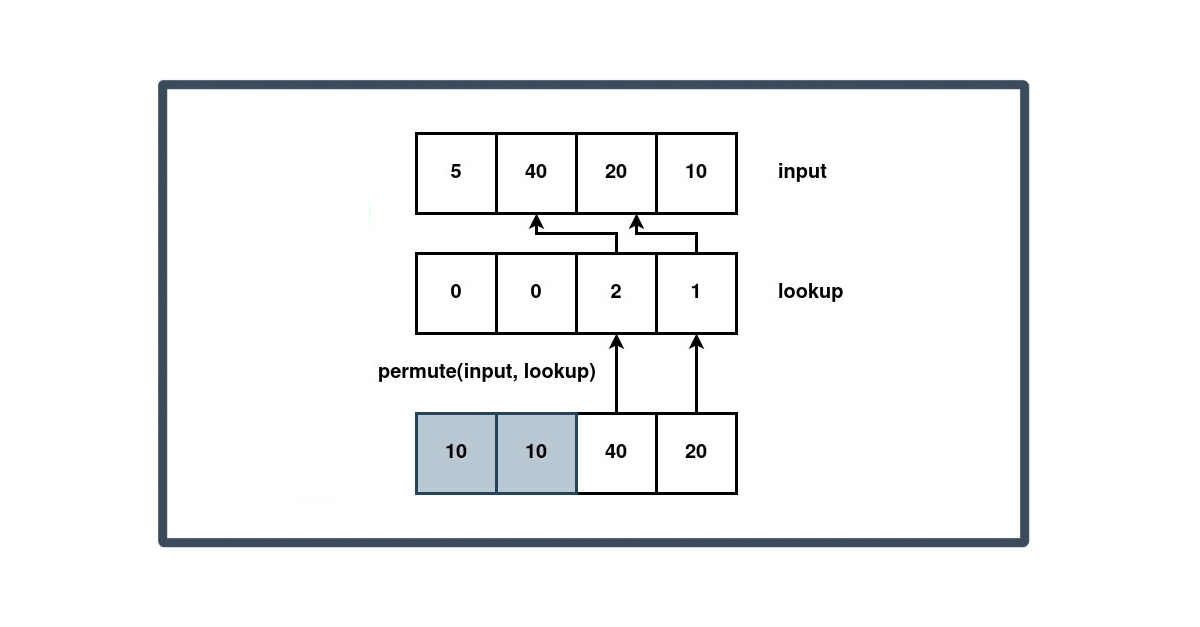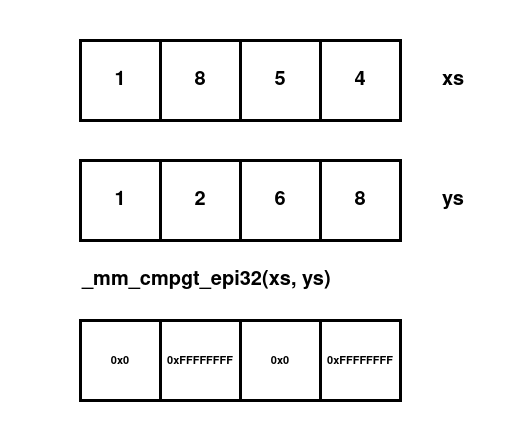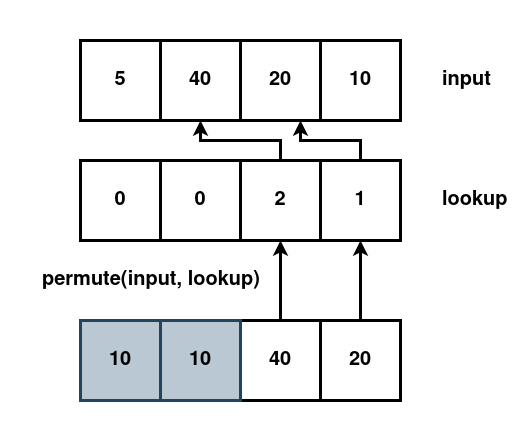December 9, 2022

# Sorting with SIMD

#### Folkert

Embedded software engineerGoogle recently published a blog article and paper introducing their SIMD-accelerated sorting algorithm.

SIMD stands for single instruction, multiple data. A single instruction is used to apply the same operation to multiple pieces of data. The prototypical example is addition, where one instruction can do e.g. 4 32-bit additions. A single SIMD addition should be roughly 4 times faster than performing 4 individual additions.

This kind of instruction-level parallelism has many applications in areas with a lot of number crunching, e.g. machine learning, physics simulations, and game engines. But how can this be used for sorting? Sorting does not involve arithmetic, and the whole idea of sorting is that each element moves to its unique correct place in the output. In other words, we don't want to perform the same work for each element, so at first sight it's hard to see where SIMD can help.

To understand the basic concepts, I played around with the ideas from the paper Fast Quicksort Implementation Using AVX Instructions by Shay Gueron and Vlad Krasnov. They provide an implementation in (surprisingly readable) assembly on their github. Let's see how we can make SIMD sort.

## rust AVX simd basics

Because we are just exploring the idea (rather than writing a production-quality implementation), we'll be using the somewhat outdated AVX family of simd instructions in this post. These are available on intel/amd processors made in the last decade, and operate on 128-bit simd values.

The following creates a 128-bit simd value consisting of four 32-bit values, each being the i32 value `42`:

``````#[cfg(target_arch = "x86_64")]
use std::arch::x86_64::*;

let same : __m128i = _mm_set1_epi32(42i32);``````

The weird functions starting with `_mm` wrap instructions and are called intrinsics. There are intrinsics for going from standard rust types to simd types:

``````let values1 = [ 1i32, 2, 3, 4 ];
let simd_value = _mm_load_ps(unsafe { values.as_ptr().cast() });``````

... and back:

``````let mut values2= [ 0i32, 0, 0, 0 ];
_mm_storeu_ps(unsafe { values2.as_mut_ptr().cast() }, simd_value);
assert_eq!(values1, values2);``````

### SIMD Compare

Given some simd values, we can use simd instructions to do work. For instance here we perform four greater-than comparisons in one instruction with the `_mm_cmpgt_epi32` intrinsic:in code:

``````let values1 = [ 1i32, 8, 5, 4 ];
let simd_value1 = _mm_load_ps(unsafe { values1.as_ptr().cast() });
let values2 = [ 1i32, 2, 6, 8 ];
let simd_value2 = _mm_load_ps(unsafe { values2.as_ptr().cast() });

let greater_than = _mm_cmpgt_epi32(current, pivot);

let mut result= [ 0i32 ; 4 ];
_mm_storeu_ps(unsafe { result.as_mut_ptr().cast() }, greater_than);
assert_eq!(result, [ 0x0, 0xFFFFFFFF, 0x0, 0xFFFFFFFF ]);``````

`false` is encoded as the integer `0` (`0x0` in hex), while `true` is represented as the 32-bit where each bit is a one (`0xFFFFFFFF` in hex).

## Quicksort

The quicksort algorithm is a recursive sort that picks an element from the input list, the pivot, and then partitions the list into those elements smaller, and those bigger than the pivot.

The algorithm then recurses on the list of smaller and bigger elements, picking a new pivot, and eventually bottoming out in a list of either 0 or 1 elements.

Quicksort normally uses constant space - the sorting happens in-place - but for this paper a linear amount of additional space is needed to temporarily store the list of elements bigger than the pivot. I also use an explicit stack rather than recursion to make sure we can handle very large inputs.

``````pub fn sort(input: &mut [i32]) {
let mut scratchpad = vec![0; input.len()];

}

fn sort_help(initial: &mut [i32], scratchpad: &mut [i32]) {
let mut stack = vec![0..initial.len()];

while let Some(range) = stack.pop() {
let (start, end) = (range.start, range.end);
let input = &mut initial[start..end];

if input.len() <= 1 {
continue;
}

// returns the number of elements less than or equal to the pivot

if n == input.len() {
// all elements smaller than or equal to the pivot
// i.e. the pivot is in the correct position in the array
stack.push(start..end - 1);
} else {
stack.push(start..start + n);
stack.push(start + n..end);
}
}
}``````

## Permute

The core of the Fast Quicksort Implementation Using AVX Instructions paper is to use the permute instruction to implement the `partition` function. With some thought, this instruction can move all elements greater than or smaller than the pivot to one side of a SIMD value.

For our case, the instruction in question is not exposed as a convenient intrinsic. So instead, we have to reach for some inline assembly. It is always fun to use a new language feature. You should probably not use inline assembly in production code, but here we're just using it for experimentation.

``````#[target_feature(enable = "avx")]
unsafe fn vperilps(mut current: __m128, mask: (i32, i32, i32, i32)) -> __m128 {

std::arch::asm!(
"vpermilps {a:y}, {a:y}, {m:y}",
a = inout(ymm_reg) current,

);

current
}

unsafe fn permute(x: (i32, i32, i32, i32), mask: (i32, i32, i32, i32)) -> __m128 {
let y = _mm_set_epi32(x.3, x.2, x.1, x.0);
}``````

The instruction inputs are two 128-bit values: the input vector and a lookup table. The output is defined as

``````output = input[mask;

so for each position in the output, we index into the lookup table. That gives an index into the input, whose value we put in the output. Because simd values are conceptually binary numbers, they "start" from the right.

``````permute((64, 32, 16, 8), (3,2,1,0)) == _mm_set_epi32(64, 32, 16, 8)
permute((64, 32, 16, 8), (0,1,2,3)) == _mm_set_epi32(8, 16, 32, 64)``````

The trick is now to determine which elements are greater than the pivot, and pick the right mask that puts these values on the right. If our input is `_mm_set_epi32(5, 40, 20, 10)` and the pivot is 15, then the values at 40 and 20 should move to the right: 20 moves to the right-most position, and 40 moves just to the left of that. The two remaining positions don't matter, but it is convenient to use zero as the value in the mask. Thus we end up with:``permute((5, 40, 20, 10), (0, 0, 2, 1)) == _mm_set_epi32(10, 10, 40, 20)``

we then write this output to the array with the greater values, and increment by the number of values that are greater, roughly

``````let greater = permute((5, 40, 20, 10), (0, 0, 2, 1));
top += 2;``````

But that moves the problem: how do we figure out what mask to use?

## Finding the lookup table

The core idea here is to precompute what to do in all scenarios: we're creating a lookup table. Let's look at some examples. Say the output of the comparison is

``0010``

Only the element at index 1 is bigger than the pivot (index 0 is the right-most bit). Therefore we want the element at index 1 of the input to move to index 0 of the output. Hence we put the value 1 at index 0 of the mask:

``(0, 0, 0, 1)``

``0100``

that means that we want the element at index 2 of the input to move to index 0 of the output:

``(0, 0, 0, 2)``

and if there are multiple, we want to preserve the order

``0110``

That means that we want the element at index 2 of the input to move to index 0 of the output:

``(0, 0, 2, 1)``

This (ugly, unoptimized) snippet generates the whole table:

``````// gives all the indices of the 1 bits in the input number
fn iter_ones(mut int: usize) -> impl Iterator<Item = usize> {
let mut i = 0;

std::iter::from_fn(move || loop {
if int == 0 {
return None;
}

let current = i;
let is_one = int & 1 > 0;

i += 1;
int >>= 1;

if is_one {
return Some(current);
}
})
}

fn generate_table() {
for i in 0..16 {
let mut row = [0, 0, 0, 0];

for (r, o) in row.iter_mut().zip(iter_ones(i)) {
*r = o;
}

println!("{:?}", row);
}
}``````

## Performance

So, is it faster? Not really. It's competitive with the rust standard library on my machine, but much slower on some other CPUs I tested it on. That is a little disappointing.

There are many reasons for this: the pivot is picked in a naive way, quicksort is not the best choice when lists are small. With some thought it is also possible to remove the second buffer, and have the program run using constant space.

There are also wider SIMD values, and newer instruction sets. The more recent AVX512 has special instructions which remove the need for a big lookup table.

## Conclusion

We have explored a non-intuitive use of SIMD instructions: speeding up quicksort. The solution uses simd intrinsics, some inline assembly, and the construction of a small lookup table. At least to me, this sort of trickery is extremely satisfying.

The google implementation is much more robust, but based on the same idea. They report being able to sort 1GB/s on a single CPU core. An extremely impressive result.

I'm very interested in using SIMD outside of number crunching. The simdjson paper is a great example of how SIMD can be used in parsing. SIMD can easily be used for searching, and with these ideas, we can also improve sorting. If you have examples of simd in non-intuitive ways, let me know!

## Stay up-to-date

Stay up-to-date with our work and blog posts?

## Related articles

March 15, 2023

### Optimizing a parser/compiler with data-oriented design: a case study

While working on the Roc compiler, we regularly dive deep on computer science topics. A recurring theme is speed, both the runtime performance of the code that we generate, as well as the performance of our compiler itself.

One extremely useful technique that we have been playing with is data-oriented design: the idea that the actual data you have should guide how code is structured.

February 11, 2022

### Optimizing Image Processing on the Edge

Over the past months, we have worked with Scailable to optimize their neural network evaluation. Scailable runs neural networks on edge devices, taking a neural network specification and turning it into executable machine code.
July 29, 2020

### Instant data retrieval from large point clouds

The Dutch government offers the AHN [](https://www.ahn.nl/) as a way to get information about the height of any specific place in the country. They offer this data by using a point cloud. That is, a large set of points with some additional meta information. With the current version of the AHN the resolution of the dataset is about eight points per square meter. This results in about 2.5TB of compressed data for the relatively small area of the Netherlands. While this is something that is not impossible to store locally, it does offer some challenges.